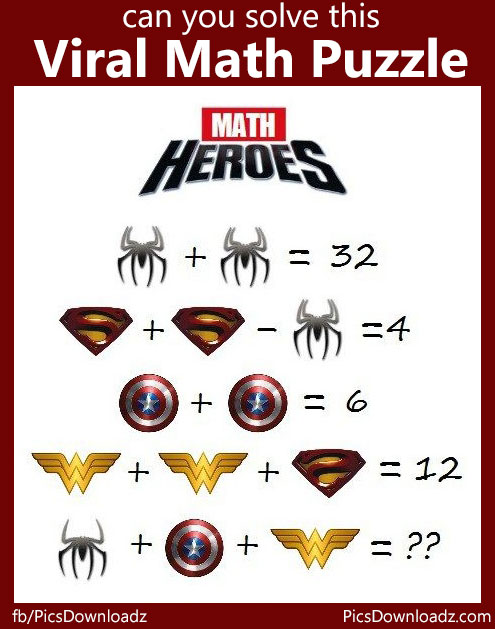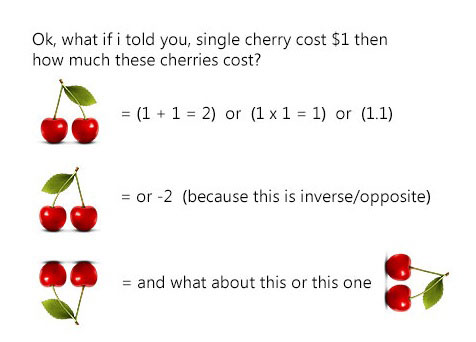This post may contain affiliate links. This means if you click on the link and purchase the item, We will receive an affiliate commission at no extra cost to you. See Our Affiliate Policy for more info.

The Viral “Math Heroes” Puzzle with Solution – Only for Genius Math Puzzles

1
4827

Can you solve this viral “Math Heroes” puzzle? Only for genius math puzzle with the solution!

Hi! Welcome back, here is another interesting puzzle on the internet which create lots of debate. You have to find out the correct value of these superheroes icon/symbols and solve the last equation.

Let’s see how’s your IQ level. Over to you!

Math Heroes Puzzle Image:Git it? Please share your answer or solution in the comment section below. Or keep scrolling for the solution.

.

A

N

S

W

E

R

.

If you got 12, 20, or 22 then you’re wrong.

According to the source: The correct answer is 26, but many have suggested 16. Let’s find out which one is correct!

Solution:

Question:

⇒ Spider + Spider = 32

⇒ Superman + Superman – Spider = 4

⇒ Captain A. + Captain A. = 6

⇒ Wonder Woman + Wonder Woman + Superman = 12

⇒ Spider + Caption A.+ Wonder Woman = ??

Let us consider:

• Spiderman = D
• Superman = S
• Captain America = C
• Wonder Woman = W

Now, solution

1) Spiderman = D

•  D + D = 32,
• 2D = 32
• D= 32/2
• D = 16

So, Spiderman = D = 16

2) Superman = S

• S + S – D = 4
• 2S – D = 4  (Put D Value from 1)
• 2S – 16 = 4
• 2S = 16 + 4
• 2S = 20
• S = 20/2
• S = 10

So, Superman = S = 10

3) Captain America = C

• C + C = 6
• 2C = 6
• C = 6/2
• C = 3

So, Captain America = C = 3

4) Wonder Woman = W

Now the debate part of the equation. If you compare the equation 2 and 4. You will figure out the differences and i’m sure everybody noticed that.

So According to the source: In this equation “S” appears inverted/Opposite, so its value is a negative number:

They consider “S” as “-S” in this equation, therefore the equation becomes:

• W + W + (S Opposite) = 12
• 2W + (-S) = 12
• 2W – S = 12
• 2W = 12 + S  (Put “S” value from 2nd equation)
• 2W = 12 + 10
• 2W = 22
• W = 22/2
• W = 11

For now, let’s solve this without “opposite S” and consider it as; S = 10, then our equation become;

• W + W + S = 12
• 2W + S = 12
• 2W = 12 – S
• 2W = 12 – 10
• 2W = 2
• W = 2/2
• W = 1

5 ) Final Equation with “S” vs “-S”:

But here is another twist:

In the last equation we see that the spider is incomplete, If we compare the last equation Spider with the first one, we figure out the differences of 8 legs Vs 6 legs spider.

If an 8-legged spider is worth 16, how much is a 6-legged spider worth?

• D ( with 8 legs) = 16
• D(with 1 leg) = 16/8
• D (with 1 leg) = 2
• therefore; D ( with 6 legs) = 6 × 2 = 12

So, for last equation, the value of Spider = D (with 6 legs) = 12

>> Last equation: (If Superman consider as “-S”, the value of Wonder Woman become i.e. W = 11)

⇒ Spider (D) + Caption A. (C) + Wonder Woman (W) = ??

⇒ D + C + W = ??

⇒ 12 + 3 + 11 = 26

>> Again Last equation: (If Superman consider as “S”, the value of Wonder Woman become i.e. W = 1)

⇒ Spider (D) + Caption A. (C) + Wonder Woman (W) = ??

⇒ D + C + W = ??

⇒ 12 + 3 + 1 = 16

Now the question arises, which one is correct? 26 or 16?

I have no dought that the puzzle is made for good intention. But this is not how it works. If 26 is the correct answer, then the most of the puzzle on the internet with such concepts make no sense.These all cherries are different? Of course not. How many times we have seen in these types of puzzle, where they use two apples, two stars, two oranges in the last equation. We go with the values, not with the situation or position.

In my perspective, it should not be like this. The value of Superman (S) should be treated as same as everywhere. It’s good to make confusion for the puzzle but I think this is not how a puzzle works. You give a particular icon/symbol/logo a value/cost which should be seen equally everywhere.

So, what you guys think? Do you go with 16 or 26? Please share your opinion.

Puzzles are fun, isn’t it? 🙂 Wait! here’s another twist, what if I told you, I flipped the wonder woman too 😉

What? Am I missing something? Have fun!

Share this puzzle with your friends, let’s see if they can figure out the hacks. Don’t forget to like us and join our puzzle group if you love these type of interesting and confusing puzzles.

Like our Page:

Source: matematicascercanas.com

Search items:

Heroes Math Puzzle, Math Heroes  Viral Puzzle, Only for genius math puzzle, Math Puzzle with solution, viral puzzle for facebook, math puzzle for WhatsApp, Difficult math puzzle, confusing math puzzle images, solve this puzzle, Fun Math Problems, math brain teasers, Superheroes logo Puzzle, logic puzzles for kids and adults.

1.mobeena